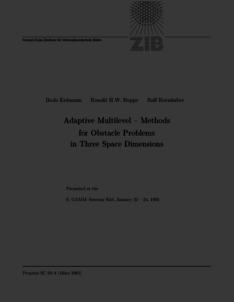Repository: Freie Universität Berlin, Math Department

# Adaptive multilevel-methods for obstacle problems in three space dimensions

Erdmann, B. and Hoppe, R. H. W. and Kornhuber, R. (1994) Adaptive multilevel-methods for obstacle problems in three space dimensions. In: Adaptive Methods - Algorithms, Theory and Applications. Notes on Numerical Fluid Mechanics (NNFM), 46 . Vieweg+Teubner Verlag, pp. 120-141. ISBN 978-3-528-07646-7Preview

545kB

Official URL: http://dx.doi.org/10.1007/978-3-663-14246-1_8

## Abstract

We consider the discretization of obstacle problems for second order elliptic differential operators in three space dimensions by piecewise linear finite elements. Linearizing the discrete problems by suitable active set strategies, the resulting linear sub-problems are solved iteratively by preconditioned cg-iterations. We propose a variant of the BPX preconditioner and prove an O(j) estimate for the resulting condition number To allow for local mesh refinement we derive semi-local and local a posteriori error estimates. The theoretical results are illustrated by numerical computations.

Item Type: Book Section Mathematical and Computer Sciences > Mathematics > Numerical Analysis Department of Mathematics and Computer Science > Institute of Mathematics 1917 Ekaterina Engel 23 Jun 2016 20:00 03 Mar 2017 14:42

Repository Staff Only: item control page